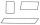# Mast

Mast has 17 m long shadow on a slope rising from the mast foot in the direction of the shadow angle at angle 9.3°. Determine the height of the mast, if the sun above the horizon is at angle 44°30'.

Result

h =  19.23 m

#### Solution:Leave us a comment of this math problem and its solution (i.e. if it is still somewhat unclear...):Be the first to comment!#### Following knowledge from mathematics are needed to solve this word math problem:

Do you want to convert length units? See also our right triangle calculator. Most natural application of trigonometry and trigonometric functions is a calculation of the triangles. Common and less common calculations of different types of triangles offers our triangle calculator. Word trigonometry comes from Greek and literally means triangle calculation.

## Next similar math problems:

1. Triangle SASCalculate the area and perimeter of the triangle, if the two sides are 51 cm and 110 cm long and angle them clamped is 130 °.
2. KitesBoys run kite on a cable of 68 meters long. What is the kite altitude, if the angle from the horizontal plane is 72°?
3. Equilateral triangleHow long should be the minimum radius of the circular plate to be cut equilateral triangle with side 19 cm from it?
4. IS triangleCalculate interior angles of the isosceles triangle with base 38 cm and legs 26 cm long.
5. DiagonalDetermine the dimensions of the cuboid, if diagonal long 53 dm has angle with one edge 42° and with other edge 64°.Between cities A and B is route 13 km long of stúpanie average 7‰. Calculate the height difference of cities A and B.
7. Trapezoid MOThe rectangular trapezoid ABCD with right angle at point B, |AC| = 12, |CD| = 8, diagonals are perpendicular to each other. Calculate the perimeter and area of ​​the trapezoid.
8. TowerHow many m2 of copper plate should be to replace roof of the tower conical shape with diameter 24 m and the angle at the vertex of the axial section is 144°?
9. RhomboidCalculate the circumference and area of the rhomboid with sides 20 and 10, with their angle 20°.
10. Regular 5-gonCalculate area of the regular pentagon with side 7 cm.
11. PentagonCalculate the area of regular pentagon, which diagonal is u=17.
12. Hexagon ACalculate area of regular hexagon inscribed in circle with radius r=9 cm.
13. Trigonometric functionsIn right triangle is: ? Determine the value of s and c: ? ?
14. RightDetermine angles of the right triangle with the hypotenuse c and legs a, b, if: ?
15. RectangleCalculate the length of the side GN and diagonal QN of rectangle QGNH when given: |HN| = 25 cm and angle ∠ QGH = 28 degrees.
16. ClimbOn the road sign, which informs the climb is 8.7%. Car goes 5 km along this road. What is the height difference that car went?The ladder has a length of 3 m and is leaning against the wall, and its inclination to the wall is 45°. How high does it reach?